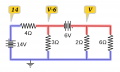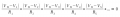# Nodal Analysis..I'm confused

#### Edmund96

Joined Oct 1, 2015
3
I've tried the question but the answer seems to doesnt agree with me .. so i think this has supernode..
(V2-14)/4 =v2/3 +v3/2 + v3/6 .....1
-v2 -6v+v3.......2

#### Attachments

• 1.2 MB Views: 4

#### shteii01

Joined Feb 19, 2010
4,644
You have three nodes. One node is reference. So you now have two nodes, that means you must have two equations.
So far I only see one equation and it is not right.

#### WBahn

Joined Mar 31, 2012
29,496
I see four nodes. One is the reference and another is trivial.

I see one equation that has the right terms (assuming that my mind reading skills are not letting me down) but does not apply KCL properly.

I see an expression (not an equation) that I think is an attempt to relate v2 to v3.

#### Edmund96

Joined Oct 1, 2015
3
Do you REALLY need a 1.2 MB file?

Me thinks perhaps not. Seems 16 kB works just fine:

View attachment 93531

Your equations have V2, v2, and v3. How are we supposed to know what those are referring to? Mind reading? Remote viewing?

Sorry.. for the 1.2 mb stuff.. it's my first time posting sorry..so it is like the v2 refers to voltage at node 2 and v3 is at node 3 ... and its under the topic of nodal analysis with voltage source so supernode is exist...as supernode lies between two non reference node.. the 6 v is in sipernode..for circuit that hasca supernode the rule is to have a support equation which i stated as 2 equation which is KVL ..for the 1 equation is the KCL equation since its involving node.. so yeah

#### WBahn

Joined Mar 31, 2012
29,496
so it is like the v2 refers to voltage at node 2 and v3 is at node 3
And how, pray tell, are we to know which node is node 2 and which node is node 3? Engineering is not about guessing!

... and its under the topic of nodal analysis with voltage source so supernode is exist...as supernode lies between two non reference node.. the 6 v is in sipernode..for circuit that hasca supernode the rule is to have a support equation which i stated as 2 equation which is KVL ..for the 1 equation is the KCL equation since its involving node.. so yeah
Okay, but your support equation needs to be an equation. You don't have an equation, only an expression.

#### Edmund96

Joined Oct 1, 2015
3
So
And how, pray tell, are we to know which node is node 2 and which node is node 3? Engineering is not about guessing!

Okay, but your support equation needs to be an equation. You don't have an equation, only an expression.
So -v2 -6v + v3 =0 ..

#### WBahn

Joined Mar 31, 2012
29,496
So

So -v2 -6v + v3 =0 ..
Yes.

I can see that you arrived at this equation by doing KVL from node 2 counter-clockwise up through node 3 and back, which is fine.

But you should also be able to immediately see, just by looking at the voltage source between node 2 and node 3, that

v3 - v2 = 6 V

or, equivalently,

v3 = v2 + 6 V

#### RBR1317

Joined Nov 13, 2010
712
This is a circuit where the Jedi super-node mind trick can be employed. Note that there are 4 nodes: one is the reference node, one forms a super-node (blue) with the reference node, and two form a super-node (red) for which the node equation is written.But not all parts of the super-node are at the same voltage. In order to keep things straight, one needs to label the super-node voltages. There is more than one way to label the super-node voltages; the diagram shows one way of doing it.In the general form of the node equation, shown above, each node voltage V_N will be replaced by the appropriate super-node voltage as labeled in the circuit diagram. That is the Jedi method for solving this circuit with a single equation.